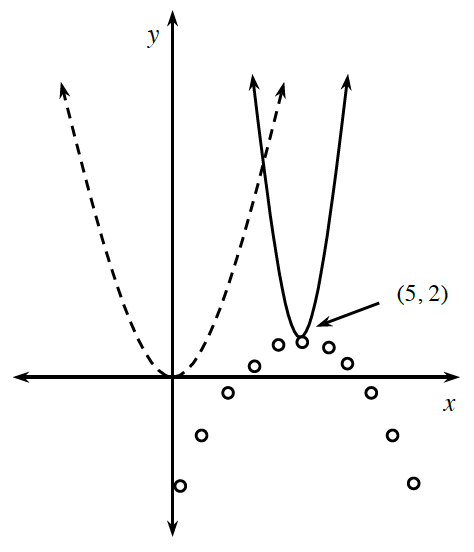### Home > CCA2 > Chapter 2 > Lesson 2.2.2 > Problem2-118

2-118.

The graph of $y=x^2$ is shown as a dashed curve at right. Estimate the equations of the two other parabolas.

Notice the location of each vertex.

Notice that one is vertically stretched and the other one is inverted and vertically compressed.

$y\approx2(x-5)^2+2, \ \ \ y\approx-\frac{1}{2}(x-5)^2+2$